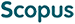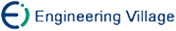李文军 王建新 陈建二. 超平面覆盖问题的参数化改进算法[J]. 计算机研究与发展, 2012, 49(4): 804-811.
 引用本文: 李文军 王建新 陈建二. 超平面覆盖问题的参数化改进算法[J]. 计算机研究与发展, 2012, 49(4): 804-811.Li Wenjun, Wang Jianxin, and Chen Jianer. An Improved Parameterized Algorithm for Hyperplane-Cover Problem[J]. Journal of Computer Research and Development, 2012, 49(4): 804-811.
 Citation: Li Wenjun, Wang Jianxin, and Chen Jianer. An Improved Parameterized Algorithm for Hyperplane-Cover Problem[J]. Journal of Computer Research and Development, 2012, 49(4): 804-811.## An Improved Parameterized Algorithm for Hyperplane-Cover Problem

• 摘要: 超平面覆盖问题是计算几何领域中一类典型的NP难问题，在实际生活中有着广泛的应用.针对NP难问题的难解性，人们提出了一些传统的方法用来求解这些NP难问题.但由于这些方法具有各自的局限性，不能满足实际应用中的各种需求，人们从新的理论角度为固定参数可解的NP难问题设计参数算法.通过深入分析直线覆盖问题(超平面覆盖问题的一个特例)的结构特征，并利用深度有界搜索树的方法，提出了一个时间复杂度为O(k\+3(0.736k)\+k+n log k)的确定性参数算法，极大地改进了当前最好的结果O((k2.2)\+2k+n log k).通过对上述算法在高维空间中的进一步扩展，提出了关于超平面覆盖问题时间复杂度为O(dk\+d+1(dk)!/((d!)\+kk!)+n\+d+1)确定性参数算法，对当前的最好结果O(k\+d(k+1)+n\+d+1)有较大改进.

Abstract: Hyperplane-cover problem is a fundamental NP-hard problem in computational geometry, which has many applications in practice. For the computational hardness of the NP-hard problems, some traditional approaches have been proposed for solving these NP-hard problems. But each of them has its own limitations, and none of them can satisfy all the application requirements in practice. Recently, a new approach dealing with NP-hard problems, called parameterized computation, has been developed, which has been effectively used in solving many hard problems. In this paper, based on the further structure analysis of the line-cover problem(a special case of hyperplane-cover problem), a deterministic parameterized algorithm with running time O(k\+3(0.736k)\+k+n log k) is proposed for the problem using depth-bounded search tree method, which significantly improves the previous best result O((k2.2)\+2k+n log k). The improvement is due to taking the advantages of the relationship between points and lines, and due to the precise algorithms running time analysis. Moreover, based on the generalization of the algorithm solving the line-cover problem in higher space, a deterministic parameterized algorithm for the hyperplane-cover problem with running time O(dk\+d+1(dk)!/((d!)\+kk!)+n\+d+1) is given, which greatly improves the previous best result O(k\+d(k+1)+n\+d+1). In particular, the algorithms proposed can be used to solve many other covering problems, such as covering points with spheres, covering points with polynomials, covering by sets with intersection at most d, etc./下载:  全尺寸图片 幻灯片
• 分享
• 用微信扫码二维码

分享至好友和朋友圈